# Gem Island

Are you fresh off the back of the long weekend and looking to sink your teeth into some combinatorics and induction? Great then you’ve come to the right place so pull your discrete mathematician stocking over your head and let’s get started.

Welcome to Gem Island! Home to `N` happy islanders. Each of which has exactly one gem. Each night something peculiar happens. One of the gems on the island is chosen uniformly at random and it splits creating a new gem for the owner. This process repeats each night for `D` nights. Here is an example of all 6 situations that could arise if there were (`N=2`) islanders after (`D=2`) nights. Here we’ve coloured the first islander’s gems red, the second green and the vertical bar separates the islanders to make things easier to read.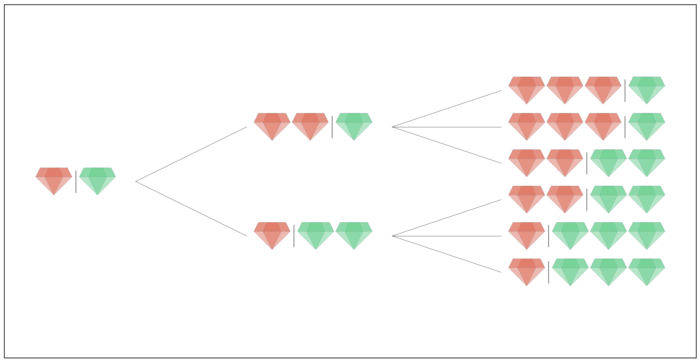We are interested in the expected value, of the number of gems, the `R` richest islanders have. In the case above the `R=1`...

# Socially Distant Polygons

Imagine a polygon, like the blue one below. Where should you stand in that polygon such you are as far as possible from your nearest vertex? This seems like a useful thing to be able to calculate in 2020 (hope this blog post ages well). Ostensibly this should be easy to compute too, but it’s not obviously that the red dot in the image below is in fact the furthest point from any of the polygon vertices.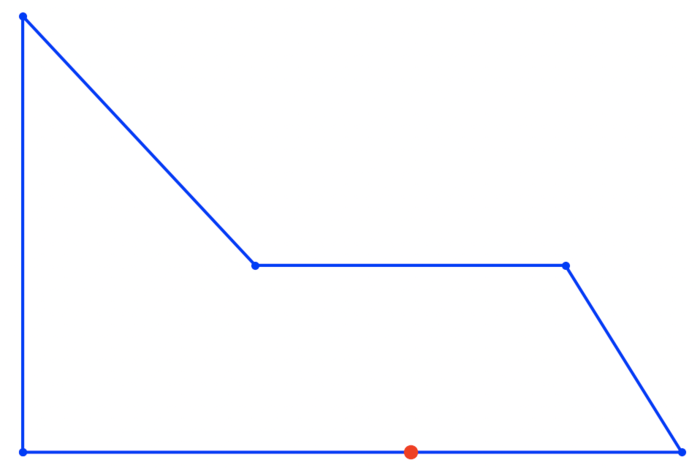A first guess might be to stand half the longest edge length away from one of the vertices. But unfortunately that doesn’t work. If you try a square you’ll see it’s best to stand in the centre which is slightly further away from each corner. This shows that we need to consider points on the interior of the polygon to make sure they are covered too. Stated another way we are trying to calculate the minimum radius circle `R`, such that the area of the union of circles positioned at `(xi,`...

# Traffic Lights

Have you ever wondered what the probability is of making it through a sequence of traffic lights without stopping? Me neither. But some people do so let’s try and solve that problem. It’s something that appears in everyday life for example my walk from home to DroneDeploy taken me through 16 traffic lights.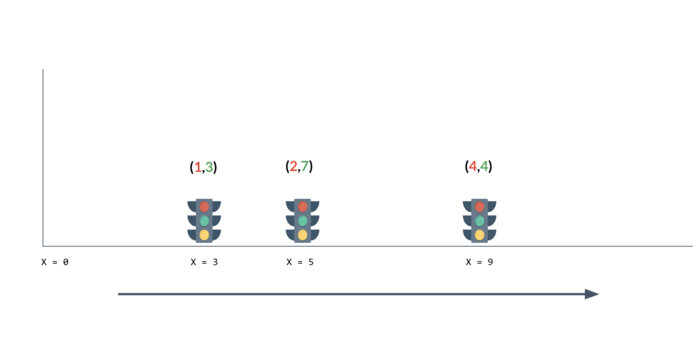Let’s say we begin at position `X=0` at time `T` in seconds and start walking as `1` meter per second right. Let’s say `T` is uniformly randomly distributed in the range `[0, 1e100]` to account for all manner of late starts. Then let’s say there are `N` traffic lines as positions `X1, X2, … ,XN` for each of these they are red for `Ri` seconds, then green for `Gi` seconds after which they repeat their cycles. At time `T= 0` all traffic lights have just turned red. So givens the lists or `X`, `R` and `G` let’s try and compute the probability that we hit each of the red lights and thus the probability that...

# Fat Albert

I was watching the Fleet Week display by the Blue Angels yesterday and we were talking about if you could determine where an aircraft was based on the sounds you were hearing from the engine.Say we have an aircraft at some unknown position flying at a constant linear velocity. If the engine is emitting sound at a constant frequency and as soon as we start hearing the engine we start recording the sound. Then given just that audio let’s try and determine how far away the aircraft is and how fast it’s traveling. Here’s a generated sample recording of a source starting `315.914` meters away and traveling at `214` meters per second in an unknown direction.

First let’s make a simplification. We can rotate our frame of reference such that the aircraft is traveling along the x-axis from some unknown starting point. If we look from above the situation looks like this.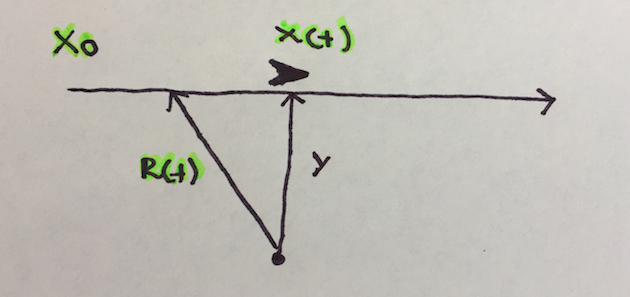When working with...

# Minkowski Asteroids

We have two convex polygons `P` and `Q` each moving at constant velocities `Vp` and `Vq`. At some point in time they may pass through one another. We would like to find the point in time at which the area of their intersection is at a maximum. Here is a simple visualization, where the yellow area represents the intersection and the arrow heads represents the velocity of the polygons.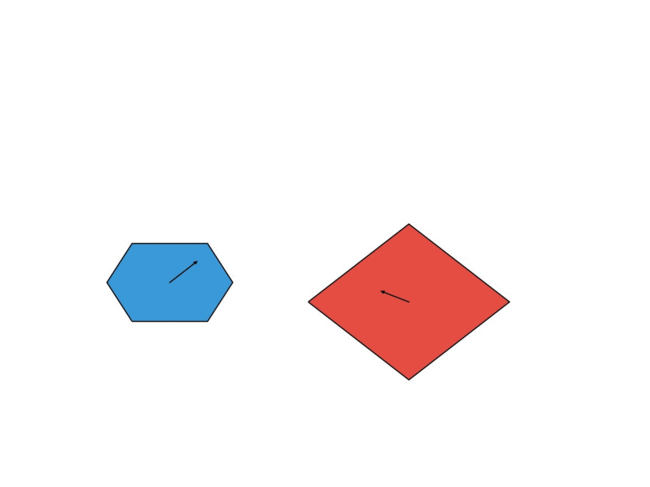Let’s first look at the problem of testing whether two polygons intersect. The simplest way to do it is to check if any edges of one polygon intersect any edges of the other. For this we need a line segment intersection algorithm. We can check if two line segments `A - B` and `C - D` intersect if the signed area of the triangle `A, B, C` is different from the signed area of the triangle `A, B, D` and similarly for `C, D, A` and `C, D, B`. It’s simple and runs in `O(N^2)`. Here’s the code:

``````import numpy as np

def area(A, B,``````
...

# Telephone Wiretapping

Imagine you are looking to intercept a communication that can happen between two people over a telephone network. Let’s say that the two people in question are part of a larger group, who all communicate with each other (sometimes via other people in the network if they don’t have their phone number). We can represent this as a graph where the vertices are people and edges connect two people if they have each other in their phone books.

Here’s a network with `6` people, some of whom don’t directly communicate with each other but can do so through others. Each person can reach all the others though, so the graph is connected.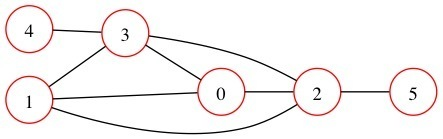Let’s also assume that this group of people communicate efficiently and use the smallest amount of calls possible and always distribute information to every person. If an unknown member of the network wants to communicate some nefarious plans to all the other...

# Geometric Cliques

If you have `N` points in the plane what is the largest subset of those points such that each point is within a distance of `D` of all the others. It seems pretty innocuous right? Turns out it’s a great big beautiful disaster.

Here’s a example of `N = 10` points where the maximum subset are the points collected with dotted lines which are each within a distance `D` of each other. There is no bigger set.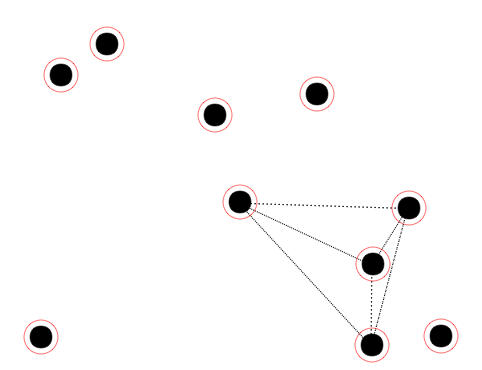Trying to compute every subset of points and checking if they are all within `D` of each other will take exponential time `O(2^N)`. So we need a better approach. Let’s try picking a point which will will assume to be part of this clique. Then all the candidate points that are not within `D` of that point won’t be in the clique. For example if we have three co-linear points spaced by `D` and select the middle one to build our clique then either the left points can be in the clique or the right one...

# Minimum Image Cover

Some applications of photogrammetry require us to collect a number of overlapping aerial images covering an area. The more overlap the better, as more overlap in pairs of images gives us a higher result in some applications. However in other applications we are actually looking for as few images as possible from the set that still cover the area of interest without any gaps.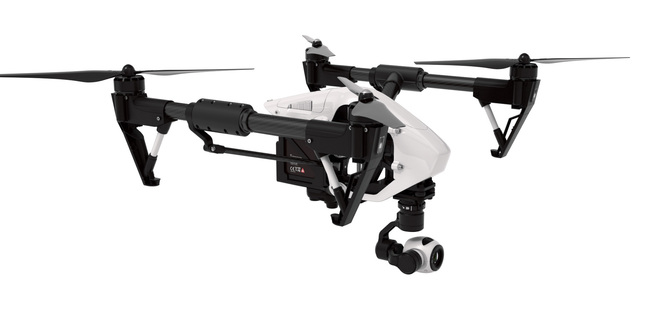Framed another way - given a collection of sets, what is the minimum number of those sets that need to be selected such that their union is the union of all of the sets. The sets in our case are defined by taking each location on the ground as a `longitude,latitude` pair and then finding the set of images that can see that location. We’ll talk about how to enumerate these locations on the ground later. This is called the set cover problem.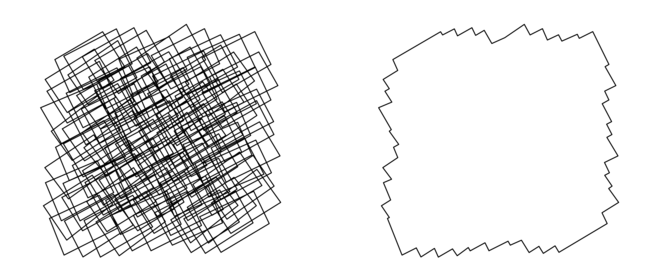## Camera Geometry

Before we start solving the problem lets generate a...

# Counting Money

This post is based on a question from the Challenge 24 competition in 2016 where we were given a photograph of each of the denominations of Hungarian currency, called Forints. In addition given a number of photos of piles of coins (some of them counterfeit) and we had to compute the total value of money automatically.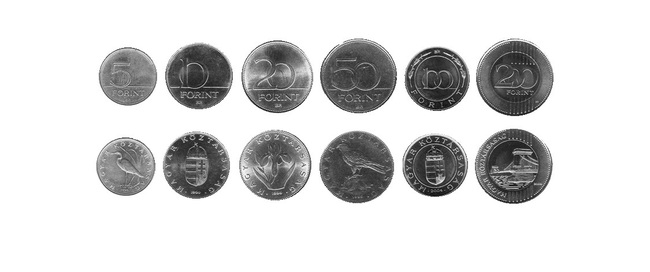First let’s look at the template and see how we can easily extract the location of the clean coins. A flood fill can compute the connected components using a BFS which runs quickly enough and since the images is quite clear we can just iterate through each unvisited non-white pixel and for each start a new component and flood out to all connected pixels that aren’t white. Here’s the code:

``````import cv2
from itertools import product

def flood_fill(img):

rows, cols = img.shape

components = {}
component_id = -1
seen = set()

for (r, c) in``````
...

# Mines Chain Reaction

This post is based on a question asked during the Challenge 24 programming competition. Given the locations of a number of land mines as `X` and `Y` coordinates and their blast radius `R`. What is the minimum number of mines that need to be detonated such that all mines are detonated. When a mine is detonated it detonates all mines within its blast radius and the process repeats.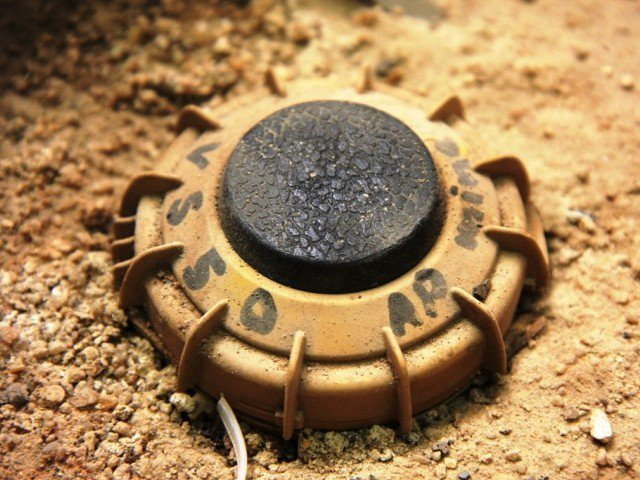Here’s a simple example with `13` mines. In this case the optimal solution is to detonate mines `0, 3` and `8` which will detonate all others. It’s not the only solution.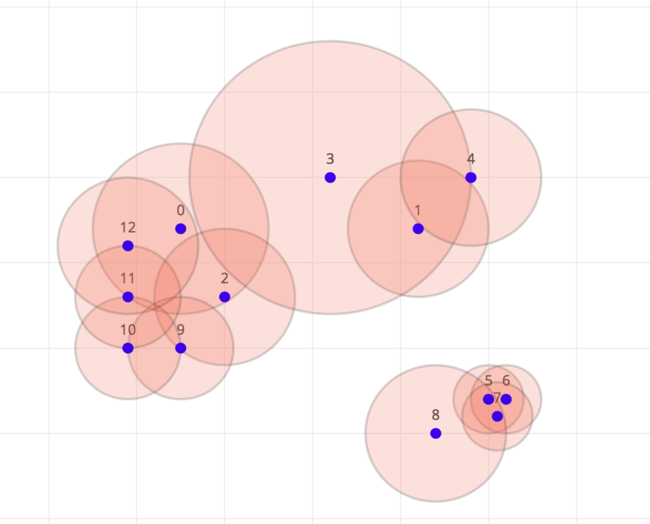The relationship between mines is not commutative. Just because mines `A` can reach mine `B` doesn’t mean that mine `B` reaches mine `A`. Therefore we can represent the mines as a directed graph where vertices are mines and there is an (unweighted) edge from mine `A` to mine `B` if mine `A` can directly detonate mine `B`.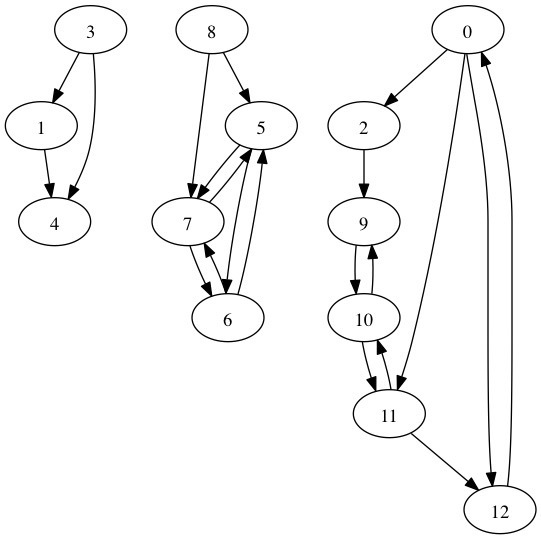In order to solve this problem we first...